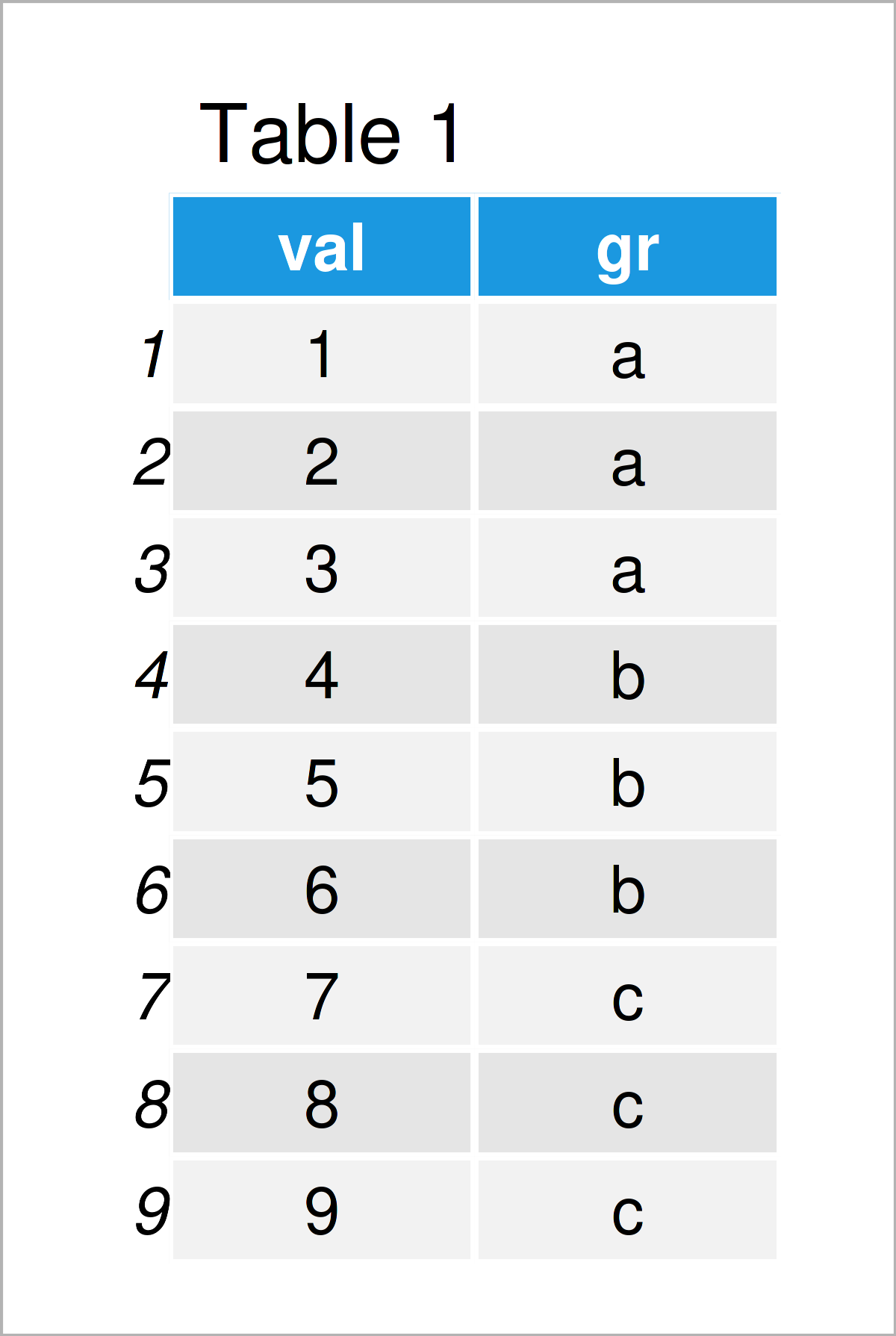# Calculate Min & Max by Group & Add as New Column in R (2 Examples)

In this R tutorial you’ll learn how to get the group minima and maxima and add the result as a new variable to a data frame.

The table of content is structured as follows:

Here’s the step-by-step process…

## Creation of Example Data

As the first step, let’s create some example data:

```data <- data.frame(val = 1:9,           # Create example data frame
gr = rep(letters[1:3], each = 3))
data                                    # Print example data frame```Table 1 illustrates the output of the RStudio console and shows that our example data consists of nine rows and two columns. The variable val is an integer and the variable gr is a character.

## Example 1: Get Minimum Value by Group & Add as Column

In Example 1, I’ll explain how to calculate the minimum value by group and how to add those values as a new column to a data frame.

For this task, we can use the ave function as shown below:

```data_min <- data                        # Duplicate data frame
data_min\$gr_min <- ave(data_min\$val,    # Calculate min by group
data_min\$gr,
FUN = min)
data_min                                # Print updated data frame```The output of the previous R programming code is shown in Table 2 – We have created a new data frame that contains the minimum value of each group as a new variable.

## Example 2: Get Maximum Value by Group & Add as Column

In Example 2, I’ll explain how to append a new column to a data frame that contains the maximum value of each group.

Once again, we can apply the ave function to achieve this:

```data_max <- data                        # Duplicate data frame
data_max\$gr_max <- ave(data_max\$val,    # Calculate max by group
data_max\$gr,
FUN = max)
data_max                                # Print updated data frame```The output of the previous R code is illustrated in Table 3 – A data frame containing the max values by group as a new column.

## Video, Further Resources & Summary

Do you want to learn more about the calculation of the group minima and maxima? Then I recommend watching the following video on my YouTube channel. I’m explaining the R programming codes of this article in the video.

Also, you may read the other articles on statisticsglobe.com:

In this article you have learned how to calculate the group min and max and append the result as a new variable to a data frame in the R programming language. In case you have further questions, tell me about it in the comments section. Furthermore, please subscribe to my email newsletter for updates on new articles.

Subscribe to the Statistics Globe Newsletter